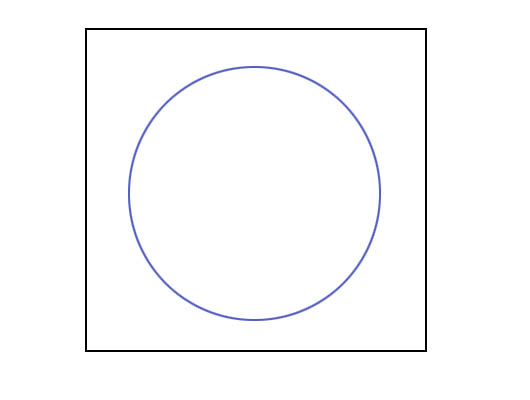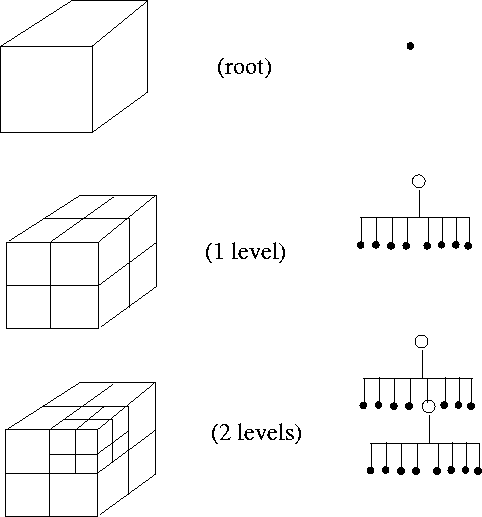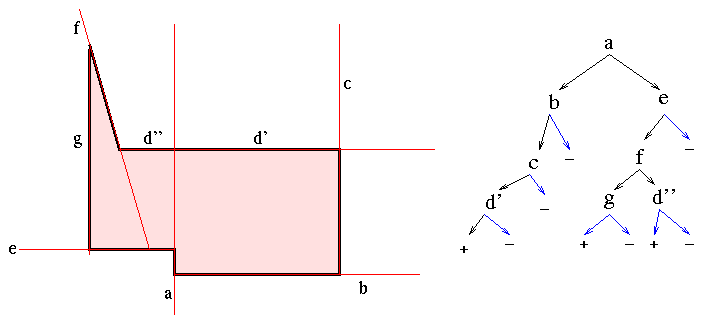# Constructive Solid-Geometry Methods

## Sweep Representation

### Specifying a 2D shape and a sweep that moves the shape through a region of space.

We perform a sweep by moving the shape along a path. At intervals along this path, we replicate the shape and draw a set of connectiong line in the direction of the sweep to obtain the wireframe reprensentation.Example of torus designed using a rotational sweep. The periodic spline cross section is rotated about an axis of rotation specified in the plane of the cross section.

Implicit/mathematical definition. Difficult to implement.

## Constructive Solid Geometry (CSG)

### 1. Definition

• Combine volume occupied by overlapping 3D objects using set boolean operations
• Each primitive is  defined as a combination of half-spaces.
• Typical standard primitives are:
cone, cylinder, sphere, torus, block, closed spline surface, right angular wedge. swept solids  (a revolution or linear sweep of a planar face which may contain holes.
• Operations are union, intersection and difference.### 2. Implementation with ray casting

Ray Casting is often used to implement CSG operation when objects are described with boundary representation. We fire a ray from the plane xy (which represent the screen). The surface limits for the composite object are determnined bu the specified set operation (see Table).Operation Surface Limit Union A, D Intersection C, B Difference (Obj2 - Obj1) B, D

This method can also be used for physical simulation.

## Visualization

The use of graphical methods to aid in analysis, often of datasets so large or complex they can not be conseptualized otherwise. The analysed data can be scalars, vectors, tensors, or a combination of these types.An example of visualization. Proteins are too complex to describe verbally

### Scalar Fields

• Graphs/charts
• Pseudo-color
• Countour plots, isolinesA topographic map of Marfa, Texas. Topographical maps are the most common utilization of isolinear plots.

### Volume Rendering

Project the data into 2D using ray casting, but the 2D value can be some function of the values along that ray.

Examples:

• Max/min values
• Accumulate the opacity of points until it reaches 1 or the ray passes through (X-rays).

The Visualization Toolkit is a cool program for doing some of these applications.

Spatial Data Structures

We will use heirarchical tree structures to store object information.

Why?

• Reduce Storage Space
• Speed up visibility computations

Let's start with 2D.

Quadtrees

### Definition

• Based on the divided-and-conquer principle, a quadtree is a data structure that recursively subdivides a plane into 4 quadrants.

• The decision to subdivide is based on an attribute of the current quadrant. If the quadrant is heterogeneous with respect to this attribute, further subdivision occurs. If it is homogeneous, or we have reached the desired level of detail, we stop subdividing.

We will apply quadtrees to storing planar polygons. Our attribute will be whether current quadrant is filled by the interior of a polygon:The succesive subdivisions can be reprensented as a tree with heterogeneous quadrants as nodes and homogeneous quadrants as leaves.

Here, we are grouping by color:F: Full ; E: Empty; P: Partially Full

For an area containing 2^n by 2^n pixels, a quadtree representation contains at most n levels.

Octrees

• Octrees are based on the same principle, but divide regions of 3D space (usually into cubes).

• The scene is subdivided at each step with three mutually perpendicular planes, aligned with the Cartesian coordinate planes.

• Individual partitions of 3D space are called Voxels (Volume Elements).

• Applications: raycasting, shadowcasting.

Octrees, like Quadtrees, use a node structure to store the volume elements:In this figure, the octree has been refined twice. First, the root is refined into eight cells, each representing an octant of the root's domain. Then, one of the root's children is refined yet again.

Here is an example of a fully subdivided torus:BSP Trees

• Binary Space-Partitiong Trees subdivide space into 2 halves at every step.

• The plane of subdivision can have any position and orientation. This reduces tree depth and search time as compared to an octree.

BSP Trees for Polygons We can represent a polygon with a BSP tree. Let each face of the polygon coincide with a partitioning plane of the tree.Now, each node of the tree encodes a face of the polygon. Each leaf of the tree is either inside or outside of the polygon.

It is easy to find out if a given point is inside the polygon; put it in the tree and see if it ends up in a + or - leaf (also have case of point exactly on a plane)

Let v be a node, p a point
point_classify( v, p )
if v is a leaf
return leaf's value ("in" or "out")
else
if p is on negative side of plane( v )
return point_classify( v.left, p )
else
if p is on positive side of plane( v )
return point_classify( v.right, p )
else (p is on the plane)
l = point_classify( p, v.left )
r = point_classify( p, v.right )
if l = r
return r
else
return "on"